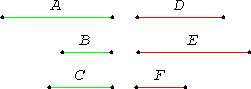# Proposition 20

If there are three magnitudes, and others equal to them in multitude, which taken two and two together are in the same ratio, and the proportion of them is perturbed, then, if ex aequali the first magnitude is greater than the third, then the fourth is also greater than the sixth; if equal, equal; and if less, less.
V.Def.18

Let there be three magnitudes A, B, and C, and others D, E, and F equal to them in multitude, which taken two and two are in the same ratio, and let the proportion of them be perturbed, so that A is to B as E is to F, and B is to C as D is to E.

Let A be greater than C ex aequali.

I say that D is also greater than F; if A equals C, equal; and if less, less.V.8

Since A is greater than C, and B is some other magnitude, therefore A has to B a greater ratio than C has to B.

But A is to B as E is to F, and, inversely, C is to B as E is to D. Therefore also E has to F a greater ratio than E has to D.

V.10

But that to which the same has a greater ratio is less, therefore F is less than D, therefore D is greater than F.

Similarly we can prove that, if A equals C, then D also equals F; and if less, less.

Therefore, if there are three magnitudes, and others equal to them in multitude, which taken two and two together are in the same ratio, and the proportion of them is perturbed, then, if ex aequali the first magnitude is greater than the third, then the fourth is also greater than the sixth; if equal, equal; and if less, less.

Q.E.D.

## Guide

The statement says that given perturbed proportions, a : b =e : f and b : c =d : e, then a >=< c implies d >=< f. The proof is straightforward.

This proposition is in preparation for V.23. Both this and V.23 rely on treating V.Def.4 as an axiom of comparability.